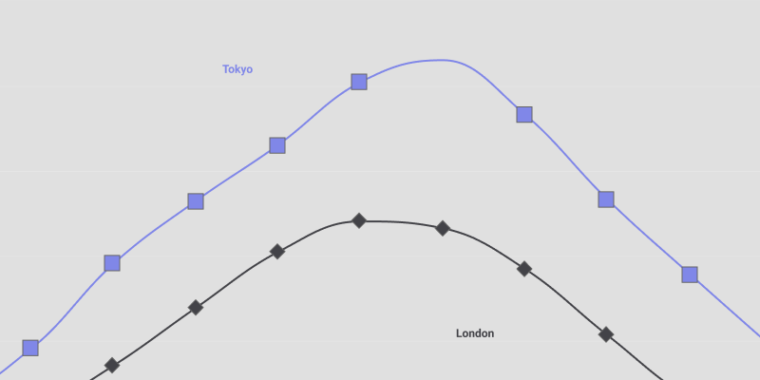# Continuous Data Type 101In the previous article, I covered the categorical data type. In this article, I explore continuous data and the mathematical tools used to analyze this data type. If you are familiar with data types and data visualization objectives, feel free to jump straight into the Chart Chooser tool to create effective charts.

Let’s begin.

Here is an excellent chart to represent the hierarchy of all data types:

Data is labeled continuous if the values are measured; for example, I have “X” kilograms of potatoes. The continuous data could be discrete (.., -1, 0, 1, 2, ..) or continuous (…, 0.123, 0,456, …).

Continuous data could be either Interval or Ratio.

## 1. Interval data

Interval data represents values where proportion doesn’t make sense, and zero has no meaning. For example, a person with 160 IQ is not twice as intelligent as a person with an IQ of 80. A temperature of 20° is not 20 times more than 0°.

Example of interval data:

• Intelligence quotient or IQ.
• Temperature.
• Time of the day, ex: 1 pm, 2 pm, 4 am, etc.

Remark
Due to the nature of the interval data, such as no meaning of proportions and zero, this data type is often used in social science: IQ, test score, income ranges, etc.

### 1.1. Statistical methods

Here are the statistical tools to explore the interval data:

• Percentiles, median, interquartile range.
• Mean, median, or mode.
• Standard deviation, range, or IQR.

### 1.2. Visualization

Line, histogram, and boxplot are the most used charts to visualize interval data since they are well suited to display statistical elements such as percentiles, median, etc.

The line chart below visualizes Tokyo and London temperature changes over 12 months.

## 2. Ratio data

For ratio data, zero has a meaning as a point of reference, and proportions make sense. For example, I can say that 4kg is two times 2kg or 4 times 1kg, and the minimum weight is 0kg.

Example of ratio data:

• Distance: 5km, 1,84m, etc.
• Weight: 81.5kg.
• Days: 2 or 3 days.
• Times: 1 hour, 34min.

Remark
Notice that ratio data does not have negative values, as there is no negative height or weight. Ratio data is popular in physical sciences.

### 2.1. Statistical methods

Here are the statistical tools to explore the ratio data:

• Percentiles, median, interquartile range.
• Mean, median, or mode.
• Standard deviation, range, or IQR.

### 2.2. Visualization

Like interval data, histogram and boxplot are used charts to visualize ratio data. The box plot demo below shows the daily company consumption 2019.

Now that you’ve read about continuous data, and I assume you also read about categorical data ;), you have an idea about how to type your data and which statistical tools and data visualizations you can use to explore it. But don’t stop here; these articles just scratch the surface. Typing your data in real-life could be challenging since the data collection process significantly influences data types.

Nevertheless, you are well equipped to continue your data science journey and ready to use the Chart Chooser tool to explore what chart can help you get the insights you need.

Let me know your comment and question in the comment section below.